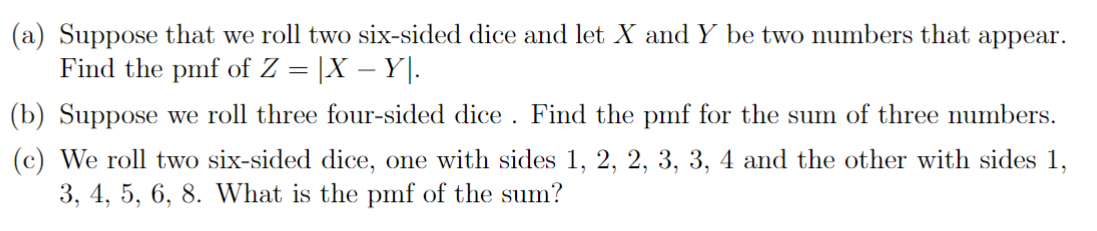Home / Expert Answers / Statistics and Probability / a-suppose-that-we-roll-two-six-sided-dice-and-let-x-and-y-be-two-numbers-that-appear-find-the-pm-pa209

# (Solved): (a) Suppose that we roll two six-sided dice and let X and Y be two numbers that appear. Find the pm ...(a) Suppose that we roll two six-sided dice and let and be two numbers that appear. Find the pmf of . (b) Suppose we roll three four-sided dice. Find the pmf for the sum of three numbers. (c) We roll two six-sided dice, one with sides and the other with sides 1 , . What is the pmf of the sum?

We have an Answer from Expert